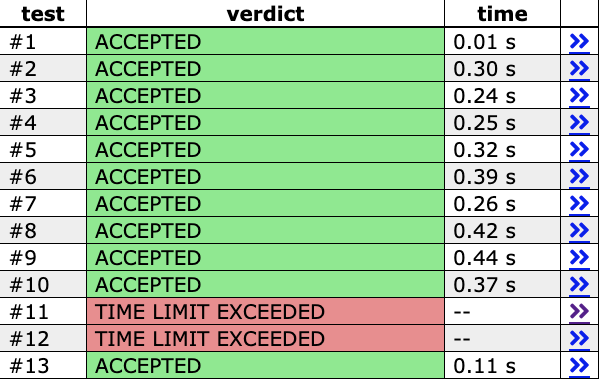# CSES Fixed-Length Paths I TLE

## Problem Info

CSES Fixed-Length Path I (https://cses.fi/problemset/task/2080/)

## My Work

#include <bits/stdc++.h>
using namespace std;

const int N = 200001;

struct L { // linked list
int x;
L *next;
} *aa[N];

void link(int i, int j) { // edge from i to j
L *l = new L();
l->x = j;
l->next = aa[i];
aa[i] = l;
};

/*
n = number of nodes
k = path length
ss[i] = i's subtree size
dep = maximum depth
cc[i] = count
*/
int n, k, ss[N], dep, cc[N]{1};
bool vv[N];
long long ans = 0;

int subtree(int x, int p = 0) { // generating ss
ss[x] = 1;
for (L *y = aa[x]; y; y = y->next)
if (!vv[y->x] && y->x != p)
ss[x] += subtree(y->x, x);
return ss[x];
}

int centroid(int dd, int x, int p = 0) { // find centroid
for (L *y = aa[x]; y; y = y->next)
if (!vv[y->x] && y->x != p && ss[y->x] >= dd)
return centroid(dd, y->x, x);
return x;
}

void _count(int x, int p, int d = 1) { // count number of other paths that have value k - length
if (d > k) return;
dep = max(dep, k);
ans += cc[k - d];
for (L *y = aa[x]; y; y = y->next)
if (!vv[y->x] && y->x != p)
_count(y->x, x, d + 1);
}

void _fill(int x, int p, int d = 1) { // fill cc up with path length from centroid
if (d > k) return;
cc[d]++;
for (L *y = aa[x]; y; y = y->next)
if (!vv[y->x] && y->x != p)
_fill(y->x, x, d + 1);
}

void decomp(int x = 1) { // centroid decomposition
int c = centroid(subtree(x) / 2, x);
vv[c] = 1;
dep = 0;
for (L *y = aa[c]; y; y = y->next)
if (!vv[y->x]) {
_count(y->x, c);
_fill(y->x, c);
}
fill(cc + 1, cc + dep + 1, 0);
for (L *y = aa[c]; y; y = y->next)
if (!vv[y->x])
decomp(y->x);
}

int main() {
cin.tie(0)->sync_with_stdio(0);

cin >> n >> k; // input
for (int i = 1, x, y; i < n; ++i) {
cin >> x >> y;
link(x, y), link(y, x);
}
decomp();
cout << ans << '\n';
}


added comments above

## What i tried

from the debugging module for TLE

1. i’ve switched from vectors to linked lists.
2. theres no infinite loops bc the code finishes running (and gets a correct answer after a minute) on my computer
3. complexity of this should be \mathcal O(N\log N) from centroid decomposition
4. i didn’t print any sterr

## Question

I looked at dolphin’s editorial (https://usaco.guide/solutions/cses-2080?lang=cpp), the code seems similar but i can’t quite find the bug.

## Checklist

See How to ask for help on a problem for more information.

• Include a link to the problem
• Format your post (especially code blocks)
• Include what you’ve tried so far
• Add comments to your code
• Read the debugging module

Any help would be appreciated! Thanks!

edit: here are the resultsDid you know that TC 11 is a line graph? (K=N-1)

#include <bits/stdc++.h>
using namespace std;

const int N = 200001;

struct L { // linked list
int x;
L *next;
} *aa[N];

void link(int i, int j) { // edge from i to j
L *l = new L();
l->x = j;
l->next = aa[i];
aa[i] = l;
};

/*
n = number of nodes
k = path length
ss[i] = i's subtree size
dep = maximum depth
cc[i] = count
*/
int n, k, ss[N], dep, cc[N]{1};
bool vv[N];
long long ans = 0;

int subtree(int x, int p = 0) { // generating ss
ss[x] = 1;
for (L *y = aa[x]; y; y = y->next)
if (!vv[y->x] && y->x != p)
ss[x] += subtree(y->x, x);
return ss[x];
}

int centroid(int dd, int x, int p = 0) { // find centroid
for (L *y = aa[x]; y; y = y->next)
if (!vv[y->x] && y->x != p && ss[y->x] >= dd)
return centroid(dd, y->x, x);
return x;
}

void _count(int x, int p, int d = 1) { // count number of other paths that have value k - length
if (d > k) return;
dep = max(dep, k);
ans += cc[k - d];
for (L *y = aa[x]; y; y = y->next)
if (!vv[y->x] && y->x != p)
_count(y->x, x, d + 1);
}

void _fill(int x, int p, int d = 1) { // fill cc up with path length from centroid
if (d > k) return;
cc[d]++;
for (L *y = aa[x]; y; y = y->next)
if (!vv[y->x] && y->x != p)
_fill(y->x, x, d + 1);
}

int work = 0;

void decomp(int x = 1) { // centroid decomposition
int c = centroid(subtree(x) / 2, x);
vv[c] = 1;
dep = 0;
for (L *y = aa[c]; y; y = y->next)
if (!vv[y->x]) {
_count(y->x, c);
_fill(y->x, c);
}
work += dep;
fill(cc + 1, cc + dep + 1, 0);
for (L *y = aa[c]; y; y = y->next)
if (!vv[y->x])
decomp(y->x);
}

int main() {
cin.tie(0)->sync_with_stdio(0);

cin >> n >> k; // input
for (int i = 1, x, y; i < n; ++i) {
cin >> x >> y;
link(x, y), link(y, x);
}
decomp();
cerr << "WORK " << work << "\n";
cout << ans << '\n';
}


work is quite huge for that test case. I’m sure you can figure out the rest on your own.

1 Like

Thanks! I figured it out; the bug was dep = max(dep, k);, and it should’ve been max(dep, d) instead.